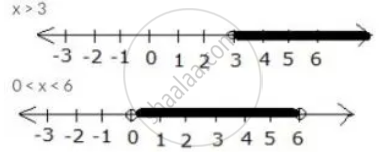Share

# Use the Real Number Line to Find the Range of Values of X for Which: X > 3 and 0 < X < 6 - Mathematics

Course

#### Question

Use the real number line to find the range of values of x for which:

x > 3 and 0 < x < 6

#### Solution

x > 3 and 0 < x < 6
Both the given inequations are true in the range where their graphs on the real number lines overlap.

The graphs of the given inequations can be drawn as:From both graphs, it is clear that their common range is
3 < x < 6

Is there an error in this question or solution?

#### APPEARS IN

Selina Solution for Concise Mathematics for Class 10 ICSE (2020 (Latest))
Chapter 4: Linear Inequations (In one variable)
Exercise 4(B) | Q: 15.1 | Page no. 49
Solution Use the Real Number Line to Find the Range of Values of X for Which: X > 3 and 0 < X < 6 Concept: Representation of Solution on the Number Line.
S